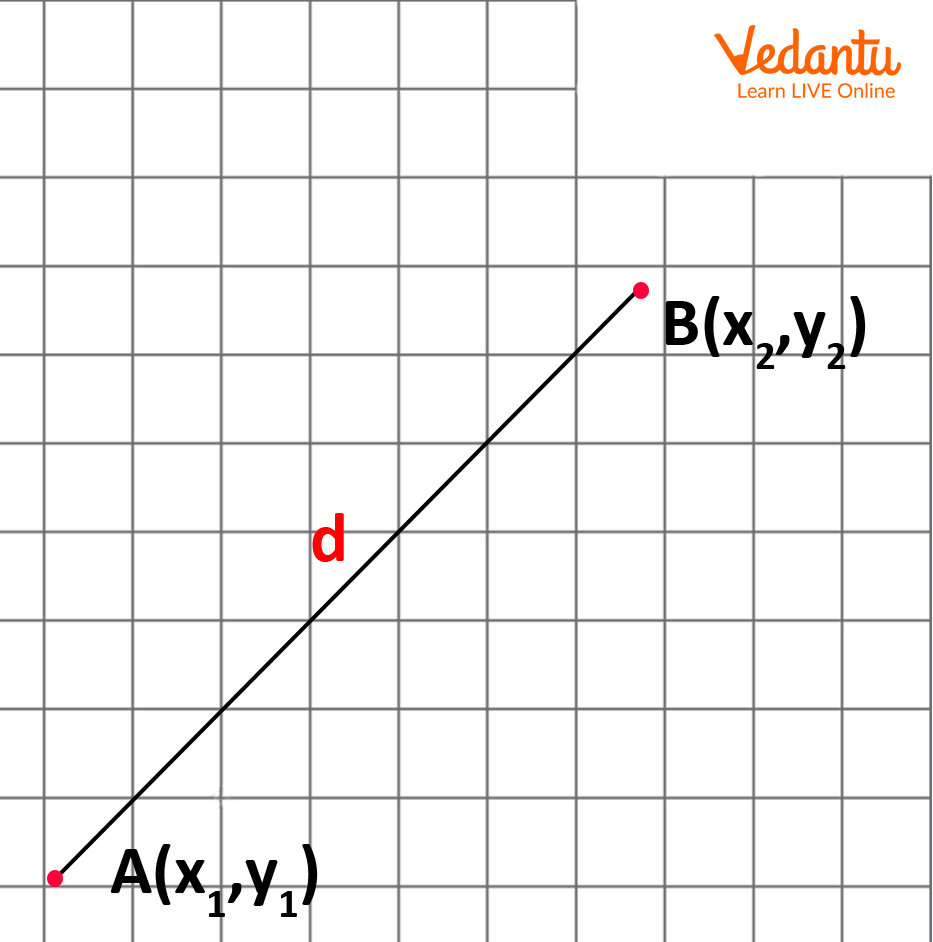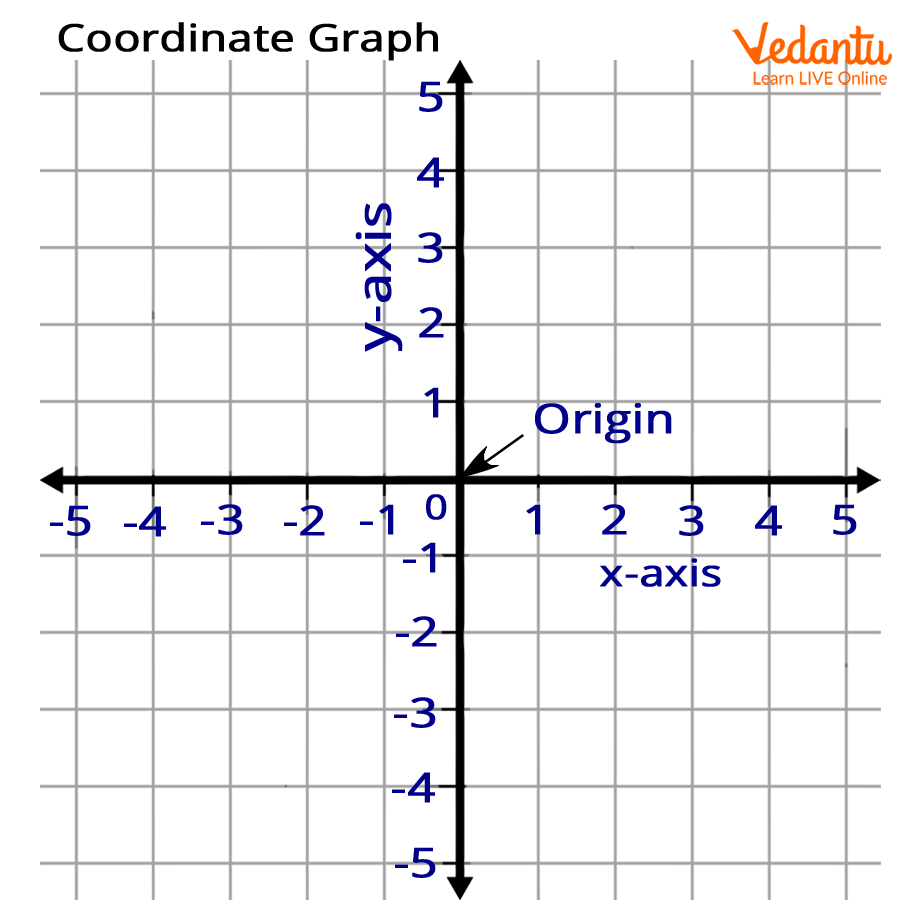Courses
Courses for Kids
Free study material
Free LIVE classes
More

# DistanceLIVE
Join Vedantu’s FREE Mastercalss

Here, you will discover how to use the distance formula to determine the separation between two points on a plane. Distance is the length that separates any two points on a line. We fix the locations of the points on the plane and their separation since we are aware of the coordinates of the two points on the plane. There is an algebraic relationship between two points' distance and coordinates.

### Distance

The length of the line connecting two places is used to measure the distance between them. The distance between two points that are on the same horizontal or vertical axis can be calculated by deducting the mismatched coordinates.Distance

### Origin

The origin is the intersection of the system axes. The origin divides each of these axes into two halves, the positive semi-axis, and the negative semi-axis. All coordinates of the origin are equal to zero that is [0,0].Origin

### One Dimensions

The bare minimum of coordinates required to identify a location in the mathematical space is known as a dimension in geometry. A one-dimensional (1D) object is one for which a point on the object may be defined using just one coordinate, according to this description.

A common definition of a 1D object is one with a length but no breadth, depth, or thickness. Lines, rays, and line segments are a few examples of geometric objects that fulfil this criterion. Rays have a single endpoint and an indefinitely long other end, while line segments have two endpoints. In the given image the two end points $A = {x_1}$ and $B = {x_2}$ are the two endpoints.One Dimensions

### Formula to Find Distance Between Two Points of One Dimensions

Suppose $A = {x_1}$ and $B = {x_2}$ are two points lying on the real number line. Then the distance between A and B is

$d(A,B) = \mid {{\bf{x}}_1} - {{\bf{x}}_2}\mid$

The distance between any two locations on the x-axis may be calculated as the absolute value of the difference between their x-coordinates because we can think of the x-axis in the plane as a one-dimensional number line. Similar to this, the absolute value of the difference in the y-coordinates of any two locations on the y-axis is the distance between them.

Now, consider the  xy-plane, and suppose ${P_1} = ({x_1},{y_1})$ and ${P_2} = ({x_2},{y_2})$ are two points in it . Then the distance between ${P_1}$ and ${P_2}$​ is

$d({P_1},{P_2}) = \sqrt {{{({x_1} + {x_2})}^2} + {{({y_1} + {y_2})}^2}}$

### Solved Examples

1. Find the distance between the points (2,4) and (4,5)

Solution: Using distance formula between the two points.

${\rm{d}} = \sqrt {{{[{\rm{y}}2 - {\rm{y}}1]}^2} + {{[{\rm{x}}2 - {\rm{x}}1]}^2}}$ ${\rm{d}} = \sqrt {{{[5 - 4]}^2} + {{[4 - 2]}^2}}$

${\rm{d}} = \sqrt {{{}^2} + {{}^2}}$

${\rm{d}} = \sqrt {[1 + 4]}$

${\rm{d}} = \sqrt 5$units

Therefore the distance between the given  points is $\sqrt 5$units

2. Find the distance of a point (0,5) from origin.

Solution: Let $[{\rm{x}}1,{\rm{y}}1]$ $= [0,5]$ and $[{\rm{x2}},{\rm{y2}}]$  $= [0,0]$

Now, Using distance formula between the two points:

${\rm{d}} = \sqrt {{{[0 - 5]}^2} + {{[0 - 0]}^2}}$

${\rm{d}} = \sqrt {{{[ - 5]}^2} + {{}^2}}$

${\rm{d}} = \sqrt {25 + 0}$

$d = \sqrt {}$

${\rm{d}} = 5$units

Therefore the distance between the given  points is 5 units.

3. Find points on the y-axis that are equidistant from  points (-1, 2) and (2, 3).

Solution: It is  known that the x-coordinate of any point on the y-axis is zero.

So  assume that the point  equidistant from these points is (0, k). that,

According to the given condition:

Distance between (0, k) and (-1, 2) = Distance between [0, k] and [2, 3]

$\sqrt {{{[ - 1 - 0]}^2} + {{[2 - k]}^2}} =$  $\sqrt {{{[2 - 0]}^2} + {{[3 - k]}^2}}$

Squaring both sides

${[ - 1 - 0]^2} + {[2 + k]^2} = {[2 - 0]^2} + {[3 - k]^2}$

$1 + 4 + {k^2} + 4k = 4 + 9 + {k^2} + 6k$

$2{\rm{k}} = 8$

${\rm{k}} = 4$

So the desired point  $[0,{\rm{k]}} = [0,4]$

### Summary

We now know that the Origin is the place where the axes converge. For each variable, the coordinates at the origin are zero. Next, we discovered how to get the formula for calculating the distance between two places. By putting your knowledge and comprehension of this subject to use and by working through worksheets on it, you may apply and improve both. The one-dimensional line contains two points at its beginning and finish, and we can use those points to calculate their distance from one another.

Last updated date: 01st Oct 2023
Total views: 65.7k
Views today: 1.65k

## FAQs on Distance

1. In arithmetic, what is the distance?

The length of the line segment connecting the two sites is used to measure the distance between them. The shortest line segment between a point and a line will have a length equal to the distance between them.

2. What's an example of distance?

The length or space between two things or people in a detached location is the general definition of distance. Four metres is an example of the distance between two street poles.

3. What is a Pythagoras Theorem?

A key concept in mathematics is the Pythagorean Theorem, which describes the relationship between the sides of a right-angled triangle. Pythagorean triples are another name for the right triangle's sides.

4. How to find the distance between the points using the Pythagorean theorem?

The distance between two points on the Cartesian plane can be calculated by applying the Pythagorean theorem. A right triangle can be constructed using a  line connecting two given  points to the hypotenuse. Here the perpendicular and the base will be straight lines parallel to the x and y axes, one end of which is one of the given points and the other end is the intersection. You can use the Pythagorean theorem ${[{\rm{hypotenuse}}]^2} = {[{\rm{base}}]^2} + {[{\rm{vertical}}]^2}$ to find the length of the hypotenuse given the  coordinates of two points. This length is equal to the distance between the two points.